Courses

# Design of Fabric Filter Computer Science Engineering (CSE) Notes | EduRev

## Computer Science Engineering (CSE) : Design of Fabric Filter Computer Science Engineering (CSE) Notes | EduRev

The document Design of Fabric Filter Computer Science Engineering (CSE) Notes | EduRev is a part of the Computer Science Engineering (CSE) Course Environmental Engineering.
All you need of Computer Science Engineering (CSE) at this link: Computer Science Engineering (CSE)

DESIGN OF FABRIC FILTER

• Pressure drop and air-to-cloth ratio are the major design parameters in bag-house design.
• Higher pressure drops implies that more energy is required to pull the gas through the system.
• Air-to-cloth ratio, also referred to as the face velocity, is the the volume flow of gas received by a bag-house divided by the total area of the filtering cloth. This ratio is the result of and is usually It is expressed as acfm/ft2. The air-to-cloth ratio determines the unit size and thus, capital cost.
• Higher air-to-cloth ratio mean less fabric, therefore less capital cost. However, higher ratio can lead to high pressure drop thus requiring higher energy. Also, more frequent bag cleanings may be required, thus increasing the downtime.
• Fabric filters are classified by their cleaning method or the direction of gas flow and hence the location of the dust deposit.

Pressure Drop: The pressure drop is the sum of the pressure drop across the filter housing and across the dust-laden fabric.

• The pressure drop across the housing is proportional to the square of the gas-flow rate due to turbulence.
• The pressure drop across the dust-laden fabric is the sum of the pressure drop across the clean fabric and the pressure drop across the dust cake.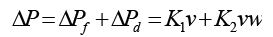(2.4.1)
Where, v=the filtration velocity; K1=the flow resistance of the clean fabric; K2=the specific resistance of the dust deposit; w=the fabric dust areal density; K1 is related to Frazier permeability, which is the flow through a fabric in cfm/ft2 of fabric when the pressure drop across the fabric is 0.5 in w.g. as follows :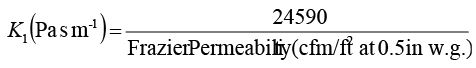(2.4.2)
Evaluation of specific resistance K2 : The dust collected on a membrane filter and K2 should be calculated from the increase in pressure drop (ΔP2- ΔP1) with filter weight gain (M2- M1) as follows: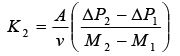(2.4.3)
Where, A is the surface area of the membrane filter.

Problem 2.4.1. A fabric filter is to be constructed using bags that are 0.1 m in diameter and 5.0 m long. The bag house is to receive 5 m3/s of air. Filtering velocity is 2.0 m/min. Determine the number of bags required for a continuous removal of particulate matter.

Solution: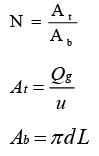Given that: Diameter of bag (d) = 0.1 m; Length of bag (L) = 5 m; Flow rate (Qg)= 5 m3/s; Filtering velocity (u)=2 m/min=0.0333 m/s.

T otal area of filter =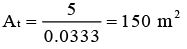Area of single bag = Ab = 3.14 x 0.1 x 5.0 = 1.57 m2

Number of bags = N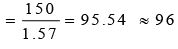The numbers of bags required for a continuous removal of particulate matter are 96.

Problem 2.4.2. A bag house is to design to handle 1000 m3/min of air. The filtration takes place at constant pressure so that the air velocity through each bag decreases during the time between clearing according to the relation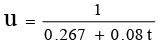Where, u is in m3/m2 min of cloth and t is time in min. The bags are shaken in sequence row by row on a 30 min cycle. Each bag is 20 cm in diameter and 3 m height. The bag house is to be square in x-section with 30 cm spacing between bags and 30 cm clearance from the walls. Calculate the number of bags required.

Solution: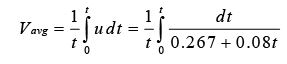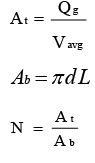Given: Ratio of flow rate air to cloth area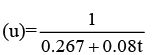( m3/m2 min of cloth). Time (t)=30 min; d- Diameter of bag (d)=0.2 m; Length of bag (L)=3 m; Flow rate (Qg)=1000 m3/min.

Average velocity (Vavg)=? (m/min)

Total area of filter (At)=? (m2).

Area of single bag (Ab)=? (m2).

Number of bags (N)=?

Put the values in equation, we get the average velocity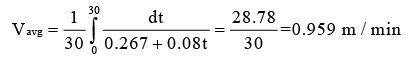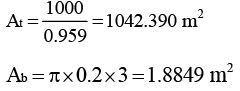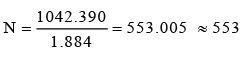The numbers of bags required for a continuous removal of particulate matter are 553.

Offer running on EduRev: Apply code STAYHOME200 to get INR 200 off on our premium plan EduRev Infinity!

## Environmental Engineering

14 videos|73 docs|70 tests

,

,

,

,

,

,

,

,

,

,

,

,

,

,

,

,

,

,

,

,

,

;